Refer to our Texas Go Math Grade 1 Answer Key Pdf to score good marks in the exams. Test yourself by practicing the problems from Texas Go Math Grade 1 Lesson 3.1 Answer Key Add 10 and More.

Essential Question
How can you use a ten frame to add 10 and some more?
Explanation:
10 + 1 = 1Explore
What is 10 + 5? Uses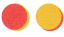and the ten frame. Model and draw to solve.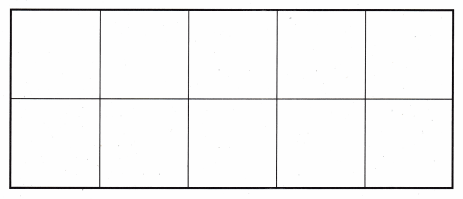Explanation: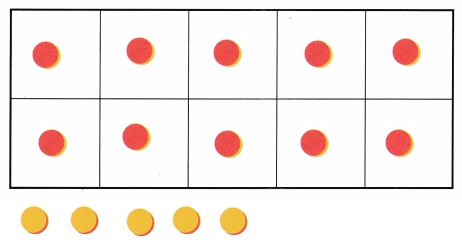For The Teacher

• Read the following problem. All has 10 red apples in a bag. She has 5 yellow apples next to the bag. How many apples does Ali have?
Explanation:
10 red apples in a bag.
5 yellow apples next to the bag.
10 + 5 = 15

Math Talk
Mathematical Processes
Explain how your model shows 10 + 5.
Explanation:
used Ten frame model for adding tens and ones.Model and Draw

You can use a ten frame to add 10 + 6.

Model and counters. Draw and color the counters to show 10 red. Draw and color the counters to show 6 yellow.
Explanation: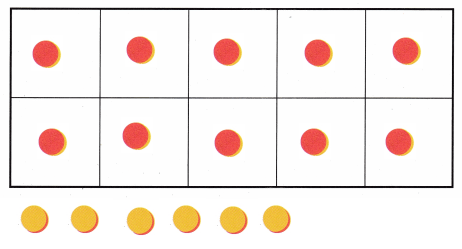10 red
and 6 yellow
10 + 6 = 16

Share and Show

Model and drawto show 10. Model and drawto show the other addend. Write the sum.

Question 1.Explanation:
Addition of tens and ones by 10 frame
10 + 3 = 13

Question 2.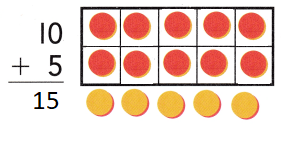Explanation:
Addition of tens and ones by 10 frame
10 + 5 = 15

Question 3.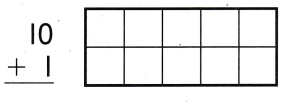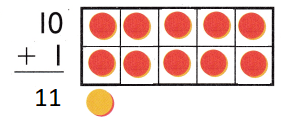Explanation:
Addition of tens and ones by 10 frame
10 + 1 = 11

Question 4.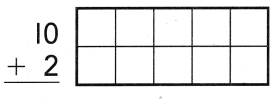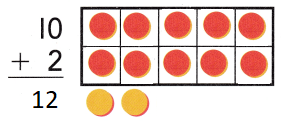Explanation:
Addition of tens and ones by 10 frame
10 + 2 = 12

Question 5.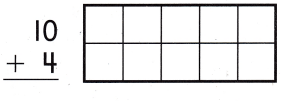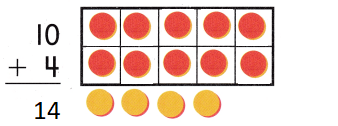Explanation:
Addition of tens and ones by 10 frame
10 + 4 = 14

Question 6.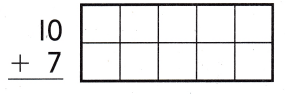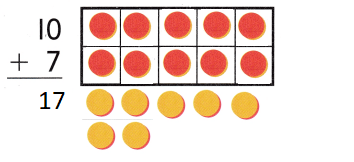Explanation:
Addition of tens and ones by 10 frame
10 + 7 = 17

Problem Solving

Model and drawto show 10. Model and draw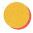to show the other addend. Write the sum.

Question 7.Explanation:
Addition of tens and ones by 10 frame
10 + 6 = 16

Question 8.Explanation:
Addition of tens and ones by 10 frame
10 + 9 = 19

Model and drawto solve. Write the addition sentence.

Question 9.
H.O.T. Marina has 10 crayons. She gets 7 more crayons. How many crayons does she have now?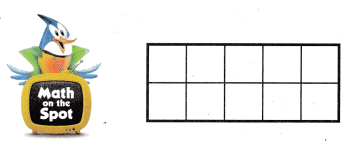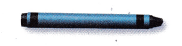___ + ___ = ___
10 + 7 = 17
Explanation:Question 10.
H.O.T. Multi-Step Austin has 10 markers. Sarah has 3 blue markers and 3 green markers. How many markers do they have?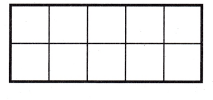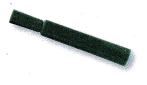___ + ___ = ___
3 + 3 = 6
10 + 6 = 16
Explanation:Question 11.
Use Tools Sara knocked down 10 bowling pins. Then she knocked down 7 more. Which shows 10 + 7?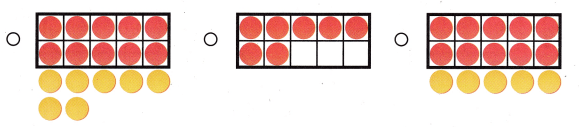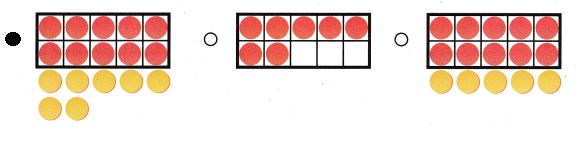Explanation:
10 + 7 = 17
Total Sara knocked down 17 bowling pins.

Question 12.
Sara’s best friend bowls two times. He knocks down 10 bowling pins the first time. He knocks down 6 bowling
pins the next time. How many bowling pins does he knock down in all?
10 + 6 = ?
(A) 16
(B) 61
(C) 7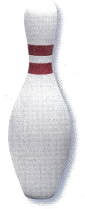Explanation:
16 bowling pins that he knock down in all
10 + 6 = 16

Question 13.
Texas Test Prep What number sentence does this model show?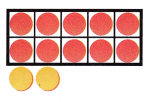(A) 10 – 2 = 8
(B) 10 + 2 = 12
(C) 5 + 2 = 7
Explanation:
The above ten frame shows
10 + 2 = 12

Take Home Activity

• Have your child choose a number between 1 and 10, and then find the sum of 10 and that number. Repeat using other numbers.

### Texas Go Math Grade 1 Lesson 3.1 Homework and Practice Answer Key

Draw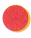to show 10. Drawto show the other addend. Write the sum.

Question 1.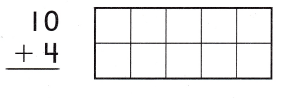Explanation:
Addition of tens and ones by 10 frame
10 + 4 = 14

Question 2.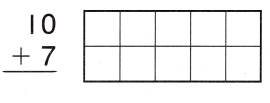Explanation:
Addition of tens and ones by 10 frame
10 + 2 = 17

Problem Solving

Draw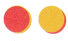to solve. Write the addition sentence.

Question 3.
Sammy picks 10 apples. Sean picks 8 apples. How many apples do they pick?
___ + ____ = ____10 + 8 = 18
Explanation:
They picked 18 apples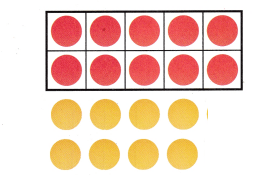Question 4.
Multi-Step Susan has 10 pears. Alex has 2 plums and 2 peaches. How many pieces of fruit do they have?
___ + ___ = ____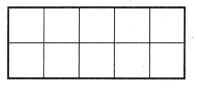10 + 2 + 2 = 14
Explanation:14 pieces of fruit do they have

Texas Test Prep

Lesson Check

Question 5.
Kayla plants 10 flowers. Then she plants 9 more. Which shows 10 + 9?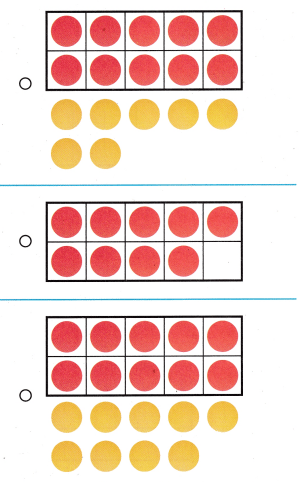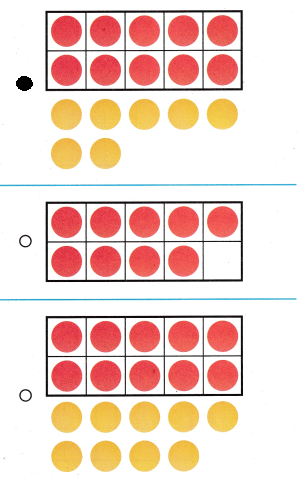Explanation:
The ten frame shows
10 + 9 = 19
The red dots show full ten frame
9 yellow show extra.

Question 6.
Multi-Step Renee plants 10 yellow flowers. Her friend Ana plants 4- red flowers and 2 orange flowers. How many flowers do they plant?
10 + 4 + 2 = ____
(A) 14
(B) 16
(C) 4